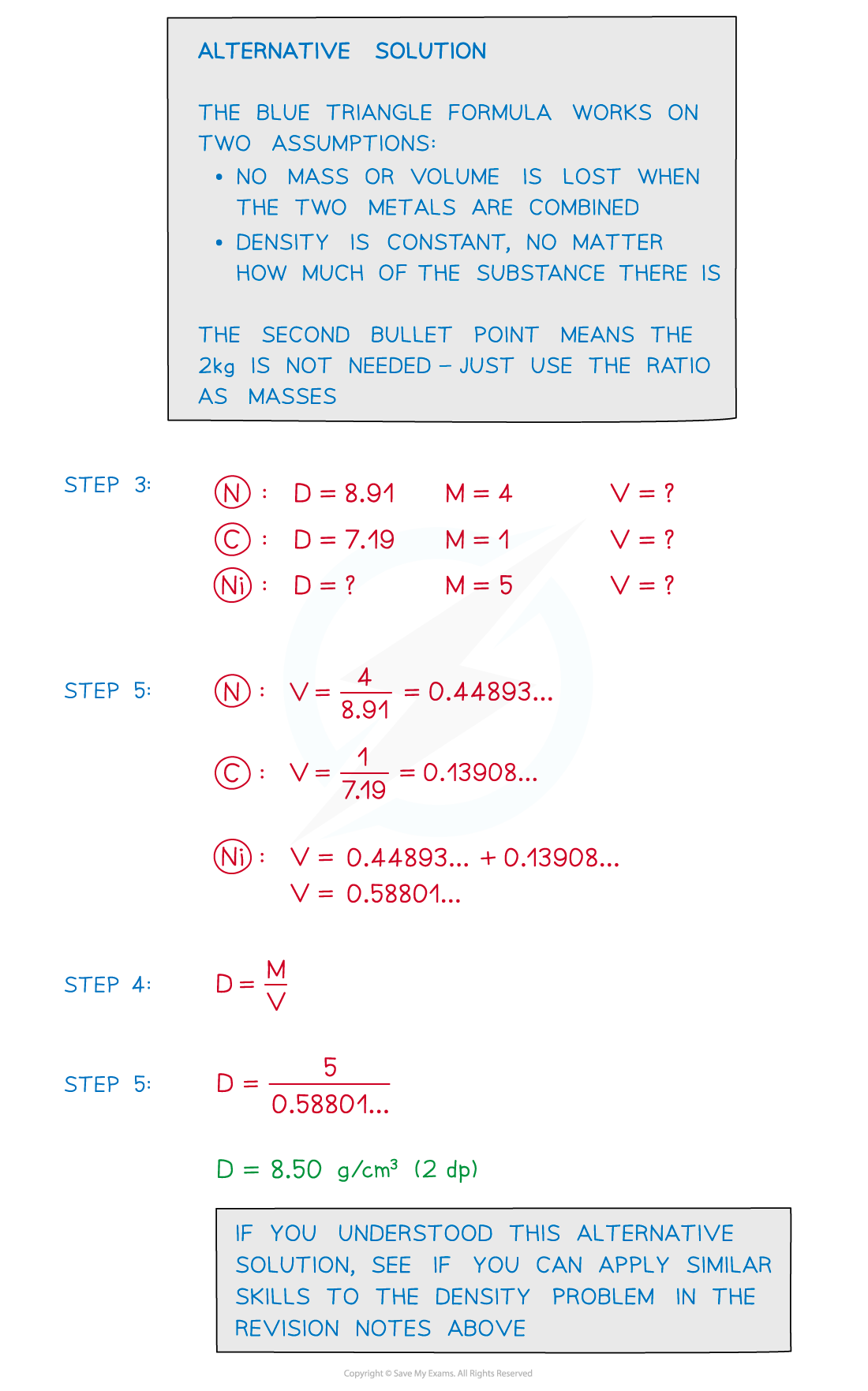# Edexcel IGCSE Maths 复习笔记 4.4.2 Speed, Density & Pressure - Harder

Edexcel IGCSE Maths 复习笔记 4.4.2 Speed, Density & Pressure - Harder

#### What are speed, density and pressure?

• Speeddensity and pressure are compound measures – they are made from other measures
• Speed is related to the measures distance and time
• Density is related to mass and volume
• Pressure is related to force and area

• The relationship between each of these sets of measures follows the same pattern – what we refer to as “blue triangles”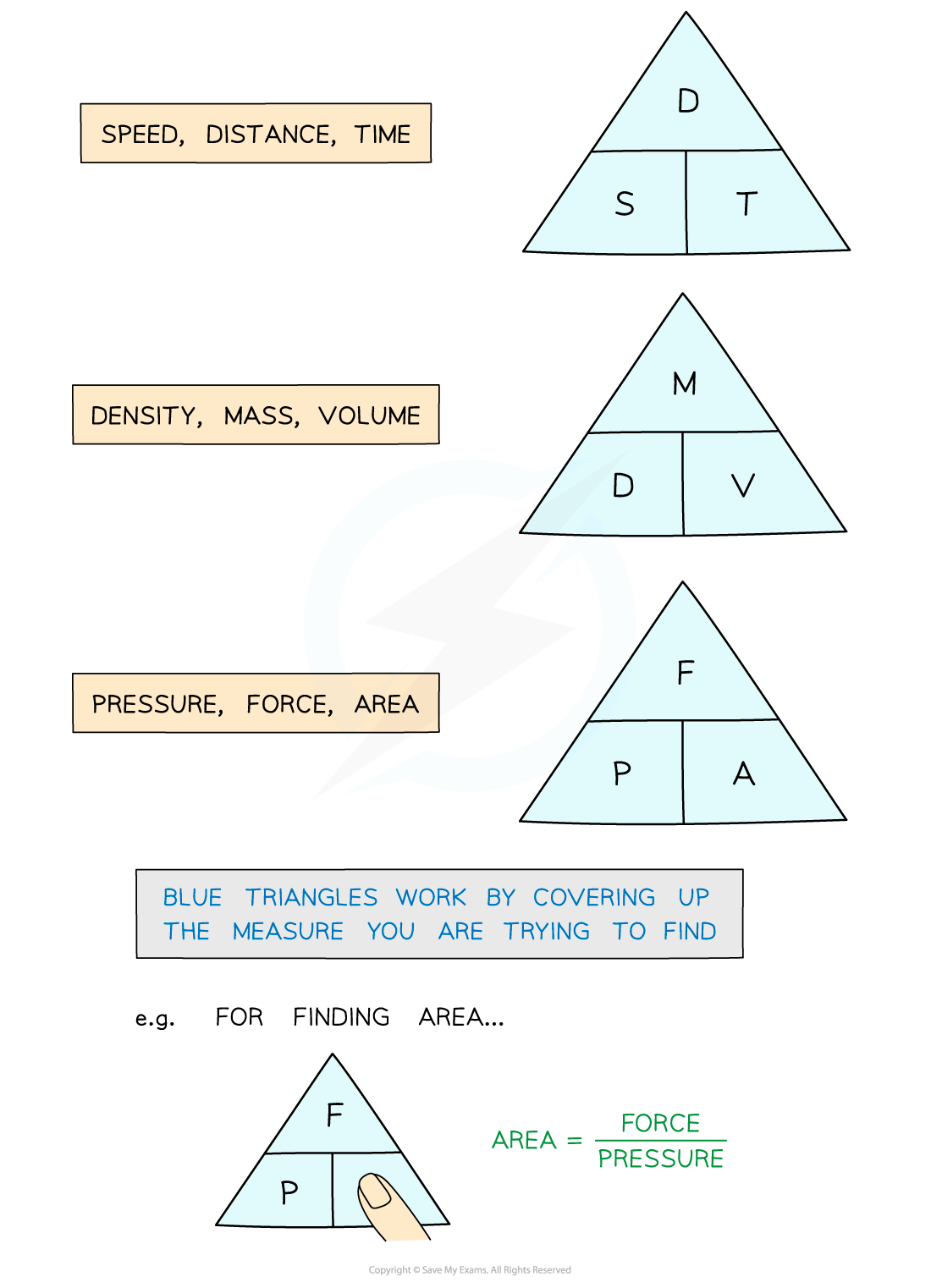• If you do not remember the blue triangles – do not worry, these can often be deduced from information given in the question – see the examples below
• It is important you understand and can do the basic questions with speed, density and pressure

#### Speed, distance and time harder problems

• Speed is commonly measured in metres per second (m/s) ormiles per hour (mph)
• There are other possibilities such as kilometres per hour (kmph)
• The units indicate speed is distance per timeie speed = distance ÷ time

• “Speed” (in this formula) means “average speed
• In harder problems there are often two journeys – or two parts to one longer journey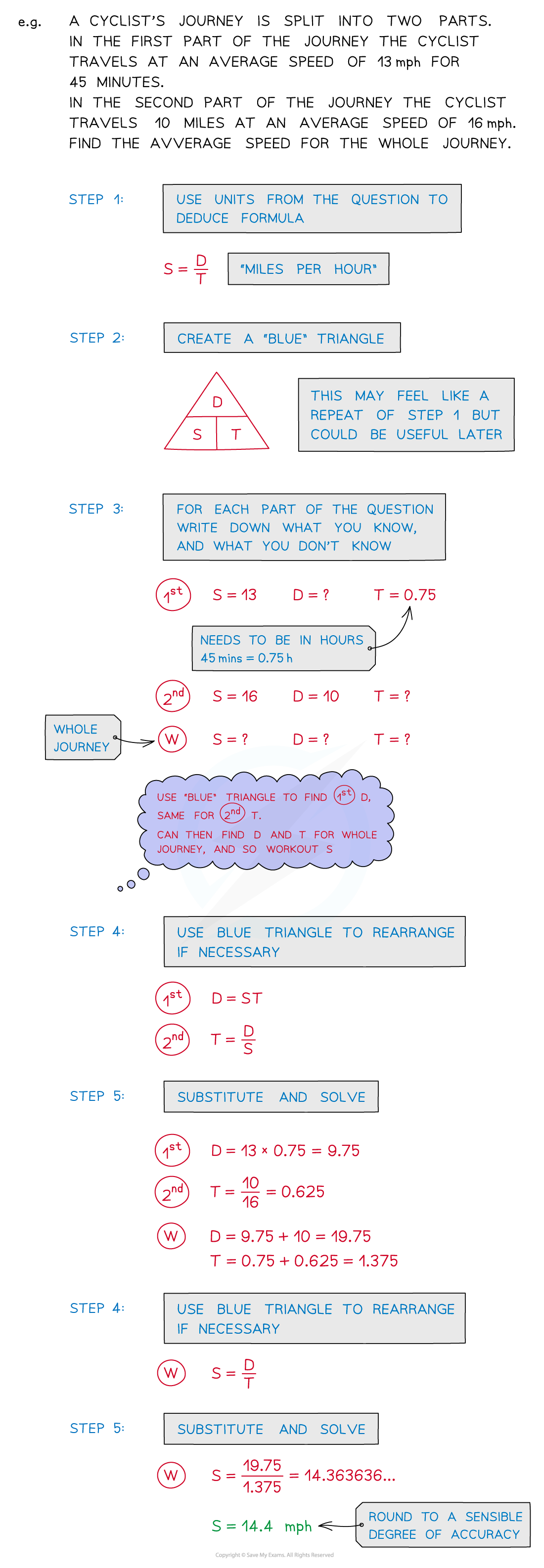#### Density, mass and volume harder problems

• Density is usually measured in grams per cubic centimetre (g/cm3)or kilograms per cubic metre (kg/m3)
• The units indicate that density is mass per volume

ie density = mass ÷ volume

• In harder problems there are often two metals (alloys), liquids or gases that have been combined rather than working with a single substance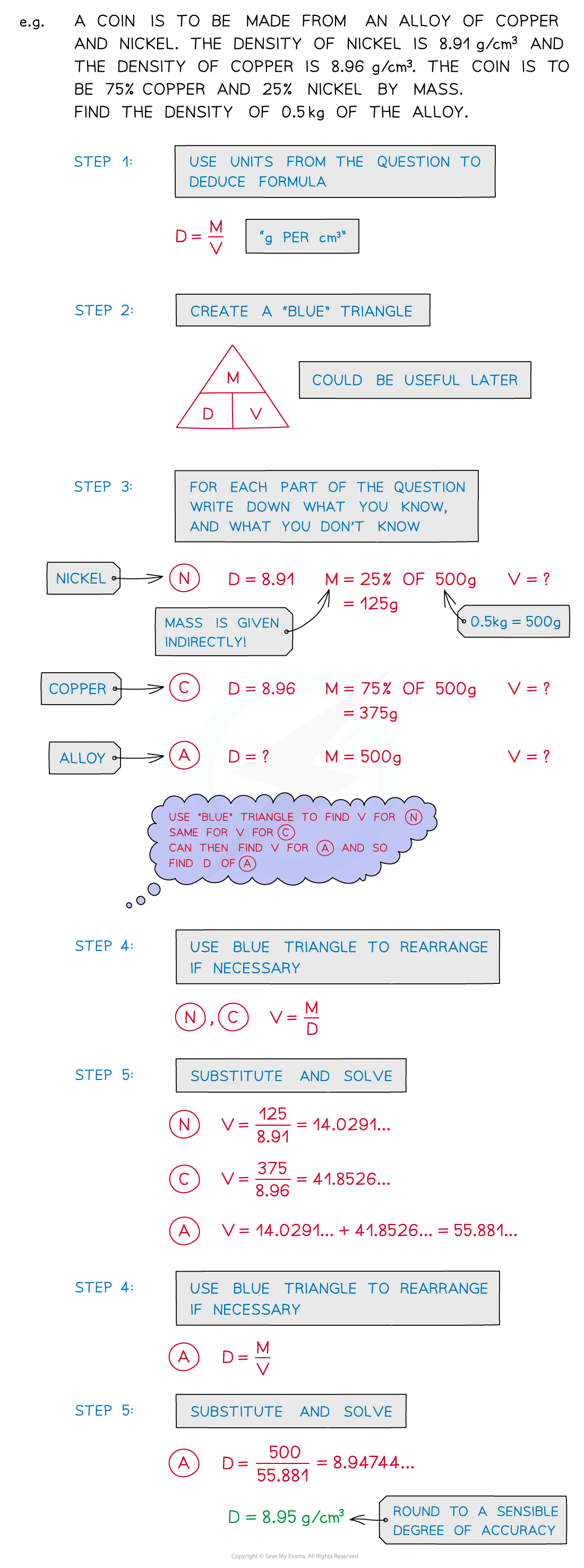#### Pressure, force and area harder problems

• Pressure is usually measured in Newtons per square metre (N/m2)The units of pressure are often called Pascals (Pa) rather than N/m2
• The units indicate that pressure is force per area

ie pressure = force ÷ area

• Remember that weight is a force; it is different to mass

#### Exam Tip

Do look out for a mixture of units:

• Time can be given as minutes but common phrases like “half an hour”(ie 30 minutes) could also be used in the same question.
• Any mixed units should be those in common use and easy to convertg to kg (and vice versa)

m to km (and vice versa)

#### Worked Example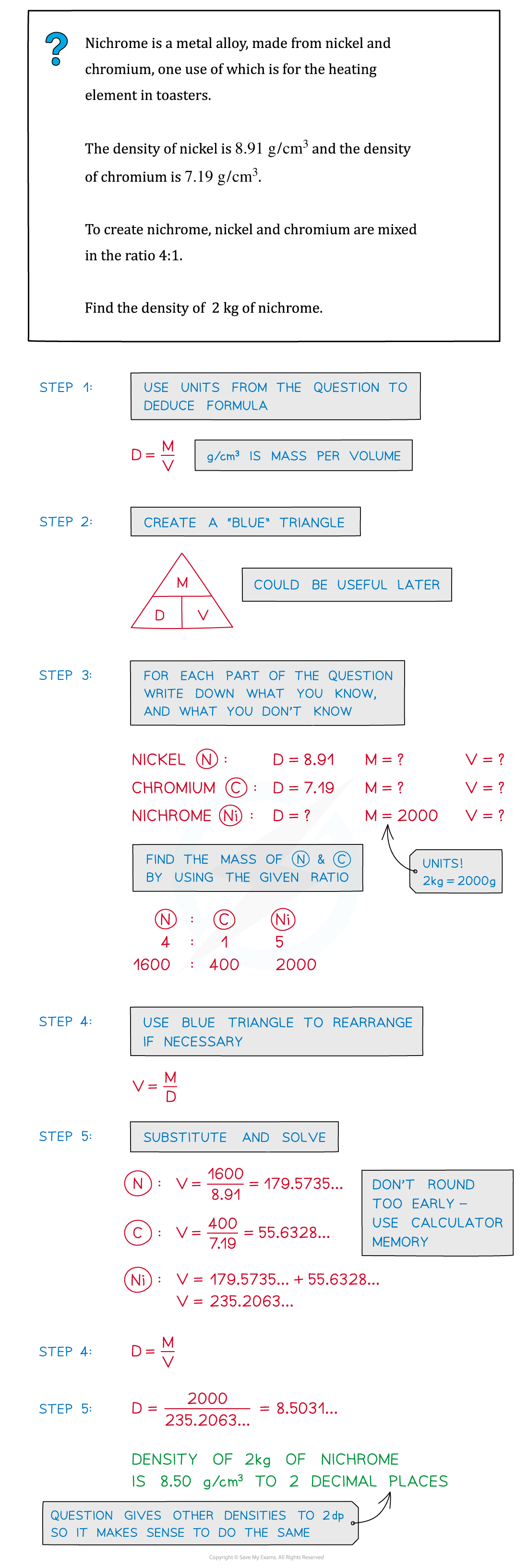#### Worked Example